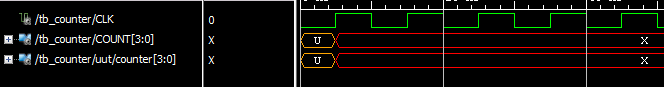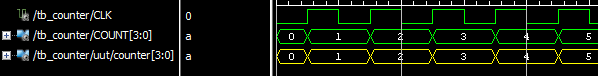# Initialising signals for simulation and synthesis

What will we see if we run a functional simulation of this VHDL counter?

1. entity top is
2. port (
3.   clk   : in std_logic;
4.   count : out std_logic_vector (3 downto 0));
5. end top;
6. architecture behavioral of top is
7.   signal counter : unsigned(3 downto 0);
8. begin
9. process (clk)
10.  begin
11.   if (clk'event and clk='1') then
12.    counter <= counter + 1;
13. end if;
14. end process;
15.   count <= std_logic_vector(counter);
16. end behavioral;

..in the simulator waveform window, the COUNT output starts at the unitialised state and then goes to the unknown state:Figure 1: Functional simulation results

But if we actually implement an FPGA with the above code, it will in fact function correctly - so why does the functional simulation not reflect this?

The counter signal is not initialised in any way, so at the start of simulation it is set to 'U' . Any operation on a signal which has a value of 'U' will always result in an unknown value, so the simulation is strictly speaking correct. So how do make it match what actually happens inside a Xilinx FPGA? One method is to initialise the value of the signal, like this:

1. signal counter : std_logic_vector(3 downto 0) := (others => '0');

If we now simulate with this modified code, we get this:Figure 2: Functional simulation with initialised signal

The counter seems to work correctly now, it starts at "0000" and increments at each rising clock edge.

Every register inside a Xilinx FPGA can be configured to have a value of either '0' or '1' after configuration, the default is '0'. By setting the initial value of the counter signal to "0000", we have made the functional match the default value of the Xilinx registers. If we want the counter to be some other value after configuration finishes, then we just modify our code:

1. signal counter : std_logic_vector(3 downto 0) := "1011";

..and now the counter starts from the value "1011" :Figure 3: Counter starts from "1011" after configuration

It is very important that the designer understands exactly how the synthesis tool that he is using will handle the initial value on the signal. The Xilinx Vivado synthesis tool will automatically ensure that the registers that are created during synthesis have the correct value after configuration.